StatLect

Sequences of random vectors and their convergence

In this lecture, we generalize the concepts introduced in the lecture entitled Sequences of random variables and their convergenceWe no longer consider sequences whose elements are random variables, but we now consider sequenceswhose generic elementis arandom vector. The generalization is straightforward, as the terminology and the basic concepts are almost the same used for sequences of random variables.Terminology

Realization of a sequence

Letbe a sequence ofreal vectors anda sequence ofrandom vectors. If the real vectoris a realization of the random vectorfor every, then we say that the sequence of real vectorsis a realization of the sequence of random vectorsand we writeSequences on a sample space

Letbe a sample space. Letbe a sequence of random vectors. We say thatis a sequence of random vectors defined on the sample spaceif all the random vectorsbelonging to the sequenceare functions fromto.

Independent sequences

Letbe a sequence of random vectors defined on a sample space. We say thatis an independent sequence of random vectors (or a sequence of independent random vectors) if every finite subset of(i.e. every finite subset of random vectors belonging to the sequence) is a set of mutually independent random vectors.

Identically distributed sequences

Letbe a sequence of random vectors. Denote bythe joint distribution function of a generic element of the sequence. We say thatis a sequence of identically distributed random vectors if any two elements of the sequence have the same joint distribution function: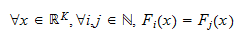IID sequences

Letbe a sequence of random vectors defined on a sample space. We say thatis a sequence of independent and identically distributed random vectors (or an IID sequence of random vectors), ifis both a sequence of independent random vectors and a sequence of identically distributed random vectors.

Stationary sequences

Letbe a sequence of random vectors defined on a sample space. Take a first group ofsuccessive terms of the sequence, ...,. Now take a second group ofsuccessive terms of the sequence, ...,. The second group is locatedpositions after the first group. Denote the joint distribution function of the first group of terms byand the joint distribution function of the second group of terms by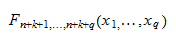The sequenceis said to be stationary (or strictly stationary) if and only if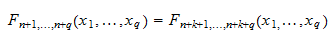for anyand for any vector.

In other words, a sequence is strictly stationary if and only if the two random vectorsandhave the same distribution (for any,and). Requiring strict stationarity is weaker than requiring that a sequence be IID (see IID sequences above): ifis an IID sequence, then it is also strictly stationary, while the converse is not necessarily true.

Weakly stationary sequences

Letbe a sequence of random vectors defined on a sample space. We say thatis a covariance stationary sequence (or weakly stationary sequence) if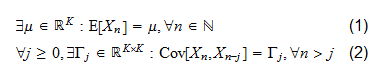whereandare, of course, integers. Property (1) means that all the random vectors belonging to the sequencehave the same mean. Property (2) means that the cross-covariance between a termof the sequence and the term that is locatedpositions before it () is always the same, irrespective of howhas been chosen. In other words,depends only onand not on. Note also that property (2) implies that all the random vectors in the sequence have the same covariance matrix (because):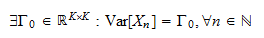Mixing sequences

The definition of mixing sequence of random vectors is a straightforward generalization of the definition of mixing sequence of random variables, which has been discussed in the lecture entitled Sequences of random variables and their convergence. Therefore, we report here the definition of mixing sequence of random vectors without further comments and we refer the reader to the aforementioned lecture for an explanation of the concept of mixing sequence.

Definition We say that a sequence of random vectorsis mixing (or strongly mixing) if and only if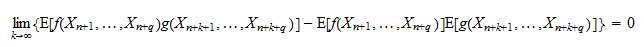for any two functionsandand for anyand.

Ergodic sequences

As in the previous section, we report here a definition of ergodic sequence of random vectors, which is a straightforward generalization of the definition of ergodic sequence of random variables, and we refer the reader to the lecture entitled Sequences of random variables and their convergence for explanations of the concept of ergodicity.

Denote bythe set of all possible sequences of realvectors. Whenis a sequence of real vectors, denote bythe subsequence obtained by dropping the first term of, that is,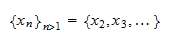We say that a subsetis a shift invariant set if and only ifbelongs towheneverbelongs to.

Definition A setis shift invariant if and only if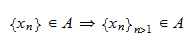Shift invariance is used to define ergodicity.

Definition A sequence of random vectorsis said to be an ergodic sequence if an olny ifwheneveris a shift invariant set.

Convergence

Similarly to what happens for sequences of random variables, there are several different notions of convergence also for sequences of random vectors. In particular, all the modes of convergence found for random variables can be generalized to random vectors:

The book

Most of the learning materials found on this website are now available in a traditional textbook format.

Glossary entries
Share